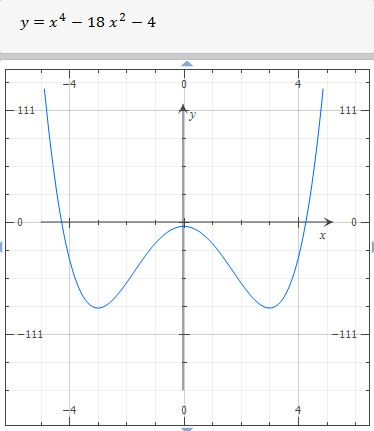# Let Q1 Q2 Q3 be the critical points of { f(x) = x^4 -18x^2 - 4 }. Let Q4 = 1 if f has a...

## Question:

Let Q1 < Q2 < Q3 be the critical points of {eq}f(x) = x^4 -18x^2 - 4 {/eq}. Let Q4 = 1 if f has a relative maximum at x = Q1, and Q4 = 0 otherwise. Let Q5 = 1 if f has a relative maximum at x = Q2, and Q5 = 0 otherwise. Let Q6 = 1 if f has a relative maximum at x = Q3, and Q6 = 0 otherwise. Then the fourth decimal of ln(3+|Q1|+2|Q2|+3|Q3|+ 4|Q4|+5|Q5|+6|Q6|) is

In 2D calculus, We can find maximum and minimum values with the first derivative test, remember the first derivative is the slope of the tangent line.

The function is:

{eq}\displaystyle \ f(x) = x^4 -18x^2 - 4 \\ {/eq}

The first derivative is:

{eq}\displaystyle \ f'(x)= 4\,{x}^{3}-36\,x \\ \displaystyle \ f'(x)= 4\,x \left( x-3 \right) \left( x+3 \right) \\ {/eq}

The function has critical values at first derivative zero:

{eq}\displaystyle \ f'(x)=0 \; \Rightarrow \; 0= 4\,x \left( x-3 \right) \left( x+3 \right) \; \Leftrightarrow \; x=-3 \; \text{ , } \; x= 0 \; \text{&} \; x=3 {/eq}

Therefore:

{eq}\displaystyle Q_1=-3 \; \text{ , } \; Q_2=0 \; \text{&} \; Q_3=3 \\ {/eq}

First derivative test:

{eq}\begin{array} \; \text{Interval} \; & { -\infty < x < -3 } & { -3 < x < 0 } & { 0 < x < 3 } & { 3 < x < \infty } \\ \hline Test \space{} value & { x = -5 } & { x = -2 } & { x = 2 } & { x = 6 } \\ Test & { f'( -5 ) = - < 0 } & { f'( -2 ) = + > 0 } & { f'( 2 ) = - < 0 } & { f'( 6 ) = + > 0 } \\ Conclusion & { decreasing } & { increasing } & { decreasing } & { increasing } \\ \end{array} \\ \therefore \boxed { \text{ increasing's interval is} \; \ ( -3 , 0 )\cup( 3 , \infty )} \\ \therefore \boxed { \text{ decreasing's interval is} \; \ ( -\infty , -3 )\cup( 0 , 3 )} \\ {/eq}Therefore,

{eq}Q_1 {/eq} is a relative minimum. Then {eq}Q_4=0 {/eq}

And,

{eq}Q_2 {/eq} is a relative maximum. Then {eq}Q_5=1 {/eq}

Also,

{eq}Q_3 {/eq} is a relative minimum, thus {eq}Q_6=0 {/eq}

Finally, the fourth decimal of

{eq}\displaystyle ln(3+|Q1|+2|Q2|+3|Q3|+ 4|Q4|+5|Q5|+6|Q6|) \; \Rightarrow \; ln(3+|-3|+2|0|+3|3|+ 4|0|+5|1|+6|0|) \; \Rightarrow \; ln(3+3+9+5) \; = \; 2.995\color{red}{7}32273554 \; \text{ is } \; \color{red}{7} {/eq}